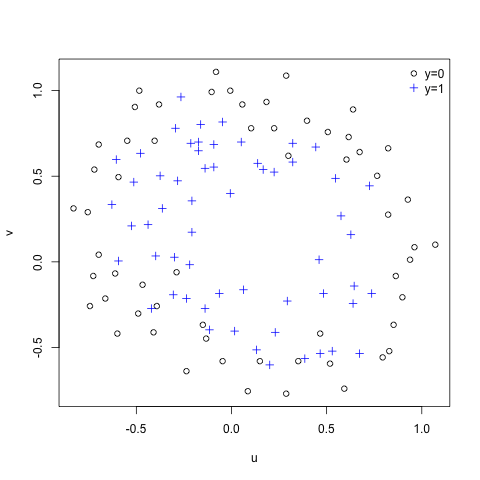Want to share your content on R-bloggers? click here if you have a blog, or here if you don't.

Exercise 5.2 Improves the Logistic Regression implementation done in Exercise 4 by adding a regularization parameter that reduces the problem of over-fitting. We will be using Newton’s Method.

## Data

Here’s the data we want to fit.

# linear regression

# plot the data
plot(mydata$u[mydata$y == 0], mydata$v[mydata$y == 0],, xlab="u", ylab="v")
points(mydata$u[mydata$y == 1], mydata$v[mydata$y == 1], col="blue", pch=3)
legend("topright", c("y=0","y=1"), pch=c(1, 3), col=c("black", "blue"), bty="n")The idea is to create a mathematical model, that separates the circles from the crosses in the plot above. And that will then allow to predict, for a new u and v value, the probability of it being a cross.

## Theory

Hypothesis is:

[ h_theta(x) = g(theta^T x) = frac{1}{ 1 + e ^{- theta^T x} } ]

The idea of the regularization is to loosen up the tight fit, and obtain a more generalized fit. For that we define the cost function, with an added generalization parameter that blunts the fit, like so:

[ J(theta) = frac{1}{m} sum_{i=1}^m [(-y)log(h_theta(x)) – (1 – y) log(1- h_theta(x))] + frac{lambda}{2m} sum_{i=1}^n theta^2] ] (lambda) is called the regularization parameter.

The iterative (theta) updates using Newton’s method is defined as:

[ theta^{(t+1)} = theta^{(t)} – H^{-1} nabla_{theta}J ]

And the gradient and Hessian are defined like so(in vectorized versions):

[ nabla_{theta}J = frac{1}{m} sum_{i=1}^m (h_theta(x) – y) x + frac{lambda}{m} theta ] [ H = frac{1}{m} sum_{i=1}^m [h_theta(x) (1 – h_theta(x)) x^T x] + frac{lambda}{m} begin{bmatrix} 0 & & & \ & 1 & & \ & & … & \ & & & 1 end{bmatrix} ]

## Implementation

Lest first define the functions above, with the added generalization parameter:

# sigmoid function
g = function (z) {
return (1 / (1 + exp(-z) ))
} # plot(g(c(1,2,3,4,5,6)), type="l")

# build hight order feature vector
# for 2 features, for a given degree
hi.features = function (f1,f2,deg) {
n = ncol(f1)
ma = matrix(rep(1,length(f1)))
for (i in 1:deg) {
for (j in 0:i)
ma = cbind(ma, f1^(i-j) * f2^j)
}
return(ma)
} # hi.features(c(1,2), c(3,4),2)
# creates: 1 u v u^2 uv v^2 ...

h = function (x,th) {
return(g(x %*% th))
} # h(x,th)

# derivative of J
G = (la/m * th)
G[1,] = 0
return((1/m * t(x) %*% (h(x,th) - y)) +  G)

H = function (x,y,th,m,la) {
n = length(th)
L = la/m * diag(n)
L[1,] = 0
return((1/m * t(x) %*% x * diag(h(x,th)) * diag(1 - h(x,th))) + L)
} # H(x,y,th,m,la)

# cost function
J = function (x,y,th,m,la) {
pt = th
pt = 0
A = (la/(2*m))* t(pt) %*% pt
return((1/m * sum(-y * log(h(x,th)) - (1 - y) * log(1 - h(x,th)))) + A)
} # J(x,y,th,m,la)


Now we can make it run, first for (lambda=1)

# setup variables
m = length(mydata$u) # samples x = hi.features(mydata$u, mydata$v,6) n = ncol(x) # features y = matrix(mydata$y, ncol=1)

# lambda = 1
# use the cost function to check is works
th1 = matrix(0,n)
la = 1
jiter = array(0,c(15,1))
for (i in 1:15) {
jiter[i] = J(x,y,th1,m,la)
th1 = th1 - solve(H(x,y,th1,m,la)) %*% grad(x,y,th1,m,la)
}


Validate that is converging properly, by plotting the Cost(J) function against the number of iterations.

# check that is converging correctly
plot(jiter, xlab="iterations", ylab="cost J")Converging well and fast, as is typical from Newton’s method.

And now we make it iterate for (lambda=0) and (lambda=10) for comparing fits later:

# lambda = 0
th0 = matrix(0,n)
la = 0
for (i in 1:15) {
th0 = th0 - solve(H(x,y,th0,m,la)) %*% grad(x,y,th0,m,la)
}

# lambda = 10
th10 = matrix(0,n)
la = 10
for (i in 1:15) {
th10 = th10 - solve(H(x,y,th10,m,la)) %*% grad(x,y,th10,m,la)
}


Finally calculate the Decision Boundary line and visualize it:

# calculate the decision boundary line
# create many points
u = seq(-1, 1.2, len=200);
v = seq(-1, 1.2, len=200);
z0 = matrix(0, length(u), length(v))
z1 = matrix(0, length(u), length(v))
z10 = matrix(0, length(u), length(v))
for (i in 1:length(u)) {
for (j in 1:length(v)) {
z0[j,i] =  hi.features(u[i],v[j],6) %*% th0
z1[j,i] =  hi.features(u[i],v[j],6) %*% th1
z10[j,i] =  hi.features(u[i],v[j],6) %*% th10
}
}

# plots
contour(u,v,z0,nlev = 0, xlab="u", ylab="v", nlevels=0, col="black",lty=2)
contour(u,v,z1,nlev = 0, xlab="u", ylab="v", nlevels=0, col="red",lty=2, add=TRUE)
contour(u,v,z10,nlev = 0, xlab="u", ylab="v", nlevels=0, col="green3",lty=2, add=TRUE)
points(mydata$u[mydata$y == 0], mydata$v[mydata$y == 0])
points(mydata$u[mydata$y == 1], mydata$v[mydata$y == 1], col="blue", pch=3)
legend("topright",  c(expression(lambda==0), expression(lambda==1),expression(lambda==10)), lty=1, col=c("black", "red","green3"),bty="n" )See that the black line ((lambda=0)) is the more tightly fit to the crosses, and as we increase the lambda values it becomes more loose(and more generalized).